Home / Tutorials / Arduino Tutorial / MQ-135 Air Quality Sensor Tutorial# MQ-135 Air Quality Sensor Tutorial

The MQ-135 “air quality” sensor is part of the MQ series of gas sensors that uses a chemical sensitive element. The resistance of such element varies when exposed to certain gases. The MQ-135 is sensitive to multiple gases including Benzene, Acetone, Alcohol and Ammonium.

### Getting Gas Concentration from MQ-135

Like all MQ gas sensors, the MQ-135 follows a sensitivity curve: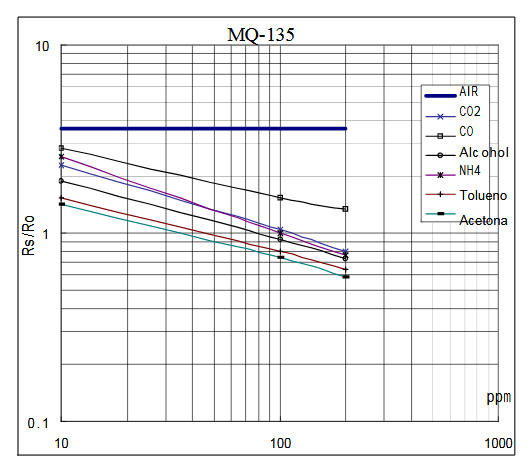The graph is a log-log graph and shows plots for multiple gases. The x-axis is the detected concentration of the gas in parts per million (PPM) while the y-axis is the RS/R0 ratio - the ratio of the sensor resistance in clean air over the resistance of the sensor in various gases.

Let’s select acetone as our target gas. There are two clear intersections of the acetone line: one is when PPM is 100 or 102 and the other is when PPM is 10 or 101:At PPM = 10, the Rs/R0 ratio is right between 1 or 100 and 1.2589 or 100.1. We can interpolate this as 100.05 hence at PPM=10, Rs/R0 should be approximately 1.122.

At PPM = 100, the Rs/R0 ratio is right between 10-0.4 and 10-0.3. This should be 10-0.35 or approximately 0.447.

Now that we have two points on the graph, we can use the two-point form of the line equation: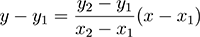We set y1 = 1.122, x1 = 10 and y2 = 0.447, x2 = 100. Our equation now for getting the concentration in PPM for acetone is: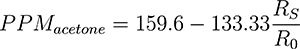The next step is to determine the resistance of the sensor in clean air R0. This can be done by measuring the output voltage of the sensor and then deriving the resistance from that voltage.

### The MQ-135 Breakout Board

For this tutorial, I am using the MQ-135 sensor breakout board:The MQ-135 breakout board, like other MQ boards, have four pins. The D0 pin goes high when sufficient levels of Benzene, Alcohol, Acetone or Ammonium are in the air. The exact concentration of these chemicals that triggers the D0 pin is unknown. Hence, measuring concentration is done through the A0 pin.

The A0 pin provides a voltage proportional to the concentration of the chemicals to which MQ-135 is sensitive. But before you can acquire the concentration in PPM, you must determine first the resistance of the sensor in clean air (R0).

### Measuring the Clean Air Resistance

Almost all MQ gas sensors follow this schematic: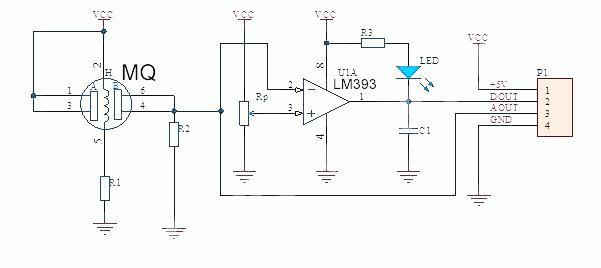Our aim is to measure the resistance of the sensor in clean air (R0). As shown, measuring the resistance between the A0 pin and GND would give us the value of resistor R2. R0 here creates a voltage divider with R2. Hence, if we can measure the output voltage at A0 in clean air, R0 is:Using an ohmmeter, measure the resistance between the A0 and GND to acquire R2. For example, the R2 on my module is approximately 1000 ohms.

Now, wire up the diagram as shown:We will be using Arduino’s analog to digital converter to determine voltage A0. Upload the sketch below:

```void setup() {
Serial.begin(9600);
}

void loop() {
// read the input on analog pin 0:
// convert to voltage:
float volts = sensorValue * 5;
volts = volts / 1023;
// print out the voltage:
Serial.println(volts);
delay(1); // delay in between reads for stability
}```

Open the serial monitor and the voltage is shown. Here’s a screenshot of my serial monitor: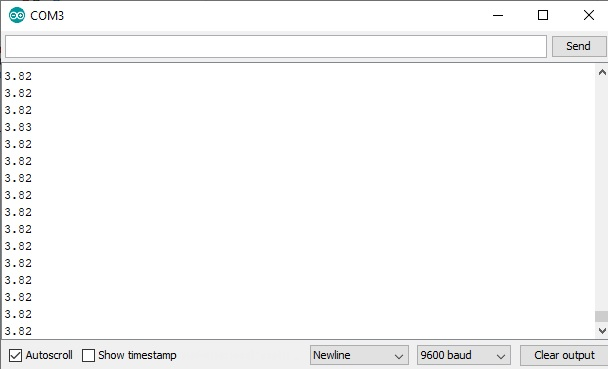Now you will notice that the value is going down. This is normal as the sensor is now preheating. Preheat time varies between MQ sensors; those which have not been used for a long time takes longer to preheat. Mine have been in storage for months, and it took almost 30 minutes to preheat. You will know it is ready when the values on the serial monitor is not drifting anymore. Mine was stable when the reading came down to 0.85.

Since I now know what my device’s A0 at clean air is, I can now determine R0 using the formula:My R2 is 1000, so that gives me an R0 of around 176 ohms.

### MQ-135 Acetone Sensor with Arduino

The concentration in acetone can now be determined using this sketch:

```int R0 = 176;
int R2 = 1000;
float RS;
float PPM_acetone;

void setup() {
Serial.begin(9600);
}

void loop() {
// read the input on analog pin 0:
// convert to voltage:
float volts = sensorValue * 5;
volts = volts / 1023;
// calculate RS
RS = R2 * (1-volts);
RS = RS/volts;
// calculate acetone PPM
PPM_acetone = 159.6 - 133.33*(RS/R0);
// print out the acetone concentration:
Serial.println(PPM_acetone);
delay(1); // delay in between reads for stability
}```

Recall that the formula for the concentration of acetone is:So we need to know Rs -- the resistance of the MQ-135 when acetone is present. This value can be acquired if we revise the formula to get R0 to:We use this value in the previous formula to get the concentration of acetone in PPM.

### Air Quality Trigger Device

Now if your project doesn’t require that the concentration of the gases is known, you can use the MQ-135 as a simple switch. We modify the wiring diagram where the D0 pin now connects to the Arduino UNO instead of the A0 pin:

This is now the sketch:

```int D0 = 2;

void setup() {
Serial.begin(9600);
pinMode(D0, INPUT);
}

void loop() {
Serial.println("Chemical detected!");
}else{;}
delay(100); // delay in between reads for stability
}```

The sketch uses the serial monitor as before. This time, it will print “Chemical detected” when any of the MQ-135 chemicals is near.

Have any questions, reactions or suggestions? Kindly drop a comment below.

## Arduino Programming: Ternary Operator

If your Arduino code involves decision-making, then it's very likely you're using conditional statements. Using …

### One comment

1.Manon van Warmerdam